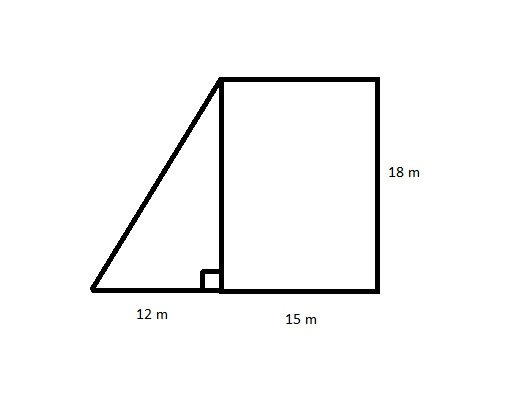# a) In 5 years, twice a puppy's current age will be equal to or greater than 15. What is the least integer that satisfies the inequality 2x+5 ge 15?Haley Madden 2022-07-24 Answered
a) In 5 years, twice a puppy's current age will be equal to or greater than 15. What is the least integer that satisfies the inequality $2x+5\ge 15?$
b) To the nearest square meter, what is the area of the figure below?c) The Philippine Trench in the Pacific Ocean is 10.05 kilometers deep. The Brazil Basin in the Atlantic Ocean is 6.12 kilimeters deep. To the nearest hundredth of a kilometer, how many kilometers deeper than the Brazil Basin is the Philippine Trench?
d) A supermarket is having a sale on canned foods. The sale includes 12 cans of soup for $\mathrm{}10.65$ . What is the unit price per can of soup to the nearest cent?
You can still ask an expert for help

• Live experts 24/7
• Questions are typically answered in as fast as 30 minutes
• Personalized clear answers

Solve your problem for the price of one coffee

• Math expert for every subject
• Pay only if we can solve itCheyanne Charles
Step 1
a) The leart integer canbe find as undear $÷$
$2x+5\ge 15\phantom{\rule{0ex}{0ex}}2x+\text{⧸}5-\text{⧸}5\ge 15-5\dots \text{Subtractiny 5 both side}\phantom{\rule{0ex}{0ex}}2x\ge 10\dots \text{Now dividing by 2 (both side)}\phantom{\rule{0ex}{0ex}}\frac{\text{⧸}2x}{\text{⧸}2}\ge \frac{\text{⧸}{10}^{5}}{\text{⧸}2}\phantom{\rule{0ex}{0ex}}x\ge 5\phantom{\rule{0ex}{0ex}}\therefore \text{The Answer is 5}$
Step 2
b) The area of the fig
The area of
triangle $=\frac{1}{\text{⧸}2}×\text{base}×\text{hei}$
$\text{i.e}=\frac{1}{\text{⧸}2}×\text{⧸}{12}^{6}×18\phantom{\rule{0ex}{0ex}}=108{m}^{2}$
Now area of rectangle $=ADEB=P×B$

We have step-by-step solutions for your answer!Urijah Estes
Step 1
c) Solve: The philippine trench = 10.05 km
The Brazil basin = 6.12 km
Therefore the philippine trench is deeper then Brasil basin by
$=10.05-6.12\phantom{\rule{0ex}{0ex}}=3.93km$
i.e 3.93 km Answer
Step 2
Solve:
Since:
Now: 12 cans of cost =1065
$\therefore =\frac{1065}{12}=8875\phantom{\rule{0ex}{0ex}}\therefore \text{one can of soup}=8875\phantom{\rule{0ex}{0ex}}\text{our 89 cent}$

We have step-by-step solutions for your answer!